output.to from Sideway
Draft for Information Only

# Content

``` Rules of Integration for Indefinite Integral  Integration by Parts for Indefinite Integral    Integration by Parts:   Techniques of Integration by Parts:```

# Rules of Integration for Indefinite Integral

In order to simplify the task of finding integrals, some integration techniques are developed to help finding integrals by transforming the integral into two parts.

## Integration by Parts for Indefinite Integral

Integration by part is method of transforming the original integral of product of two functions into two integrals for taking the advantage of substitution or transformion of the integral to an easier form to continue the integration. The strategy is to simplify the integral by transforming the original integral into two parts which allowing the integration to be continued in other easier way. The technique is making use of the Rule of Product of Functions in differentiation. i.e.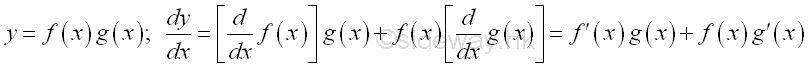Imply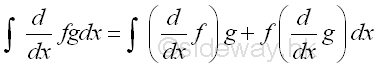### Integration by Parts:

The strategy of the method is making use of Rule of Product of Functions in differentiation for transforming the integral to two suitable formats to continue the integration.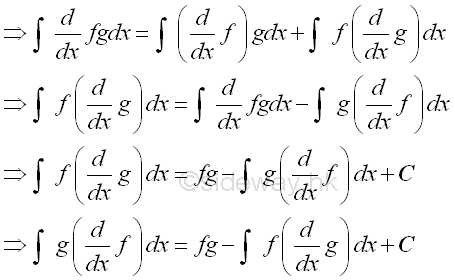As in reverse substitution, the formula is assumed the substitution is already there, but through the formula of transformation, the assumed substitued function is changed from the first function to the second or vice versa. In other words, the assumed substitued function becomes selectable after the transformation processes. The two processes of transformation are: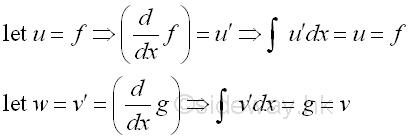Substitute back. Imply: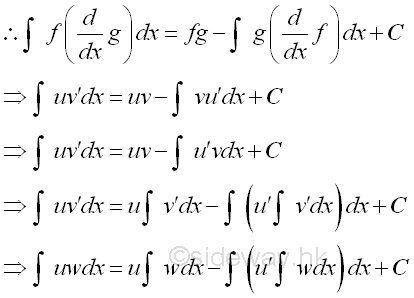The two functions of the original integrand are interchangable because of the cummutative property of function multiplications. The strategy of the method is making use of the applying of the differentiation operation to one function and the integration operation to the other function in the original integrand so that the integration of the transformed integrand can be continued.

Besides through variable of integration transformation, the formula can be applied for single function also and therefore the integration by parts can be used as formula for the interchanging of variable of integration, imply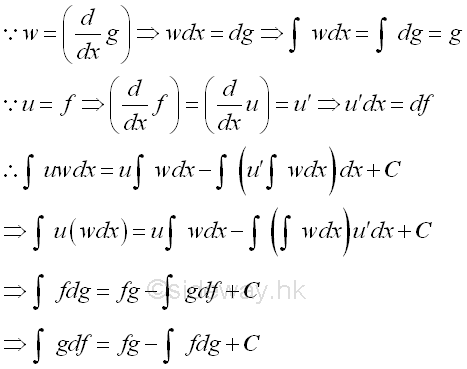### Techniques of Integration by Parts:

1. Single Function.

Method of integration by parts can also be applied for single function by assuming unity as the second function. Imply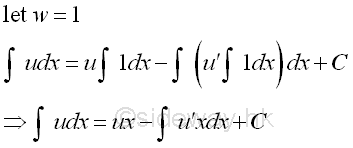Sometimes the creation of x may help to continue the integration process.

2. Integrand with one function can not be integrated by ordinary means and one function can be integrated by normal means.

Let u be the function which cannot be integrated by ordinary means. And let w be the function that can be integrated by nomral means. ImplyThis is the basic idea of integration by parts of making the integration can be continued.

3. If both functions of Integrand are integratable, and one of the function is a function of powers of x, in which the power is positive integer.

Let u be the function of power of x. And let w be the other function. Imply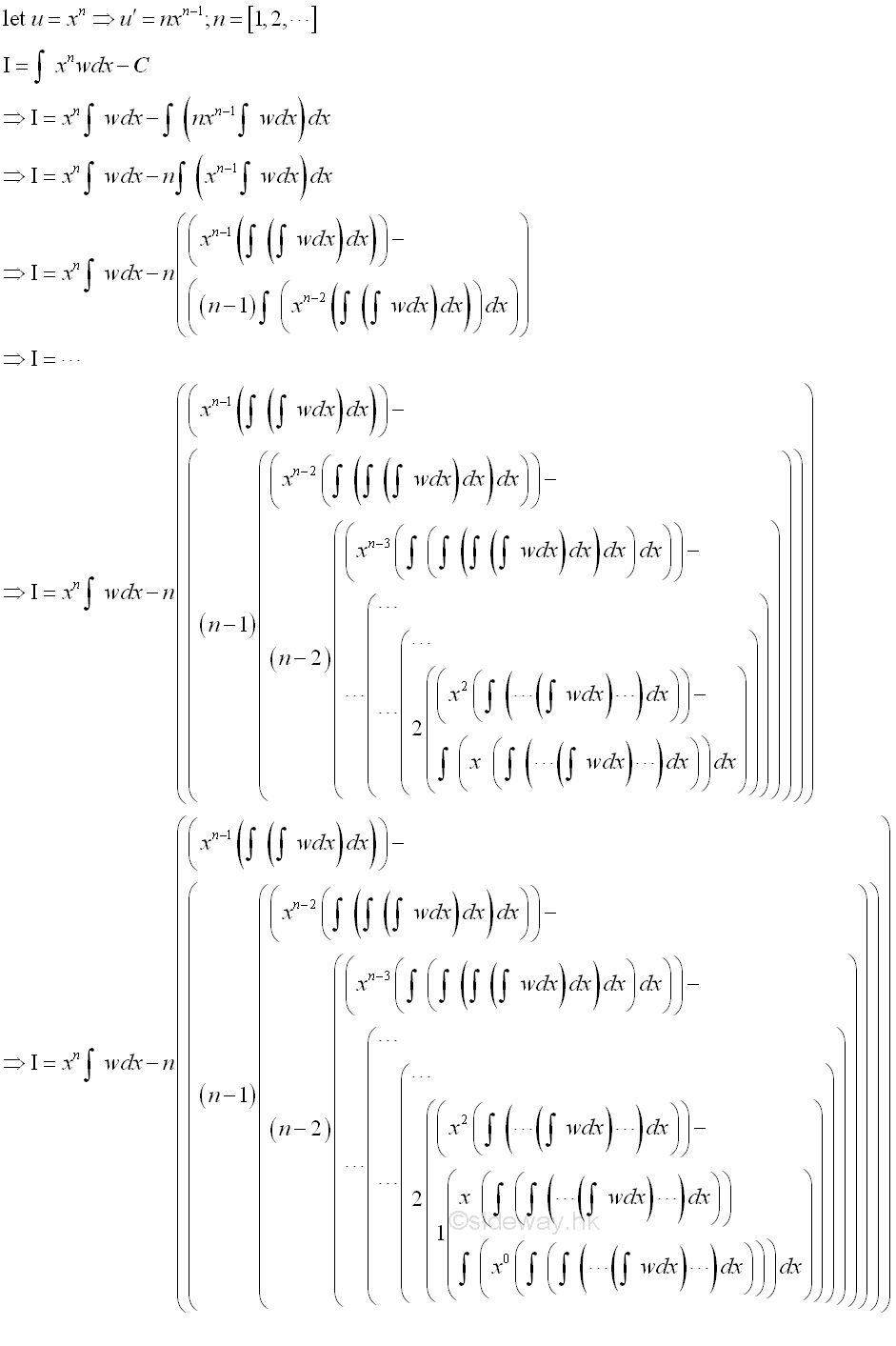The function of power of x with positive integer can be eliminated by repeating the application of integration by parts process.

4. If both functions of Integrand are integratable, and one of the function is a function of powers of sin x or cos x.

The power of  sin x or cos x can be converted using basic trigonometric identities into linear algebraic expression in terms of sin and cos that the integration by parts can be applied. The basic trigonometric identities are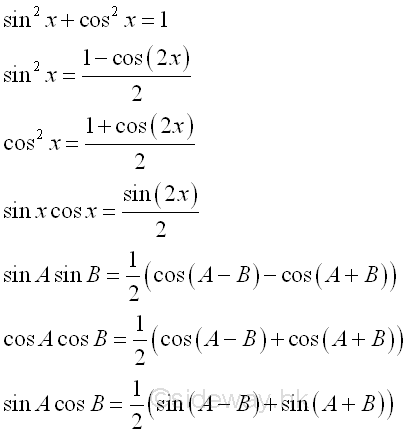After thAfter the convertion, the function with powers of sin x or cos x become integratable.

5. If the original integral occurs in the result of integration by parts, then the integral can be obtained by algebraic methed indirectly.

Sometimes the original integral occurs in the result of integration by parts after repeatedly using integration by parts, imply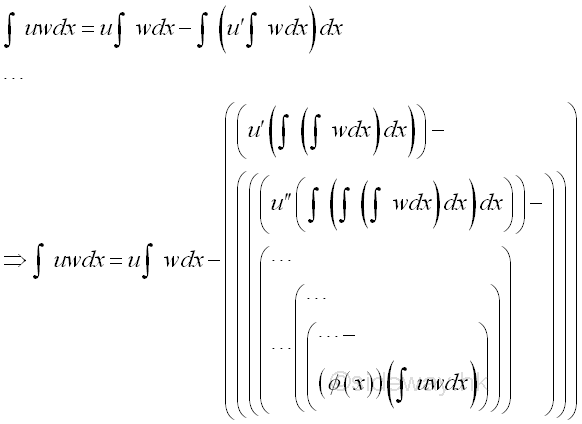Therefore the integral can be obtained by algebraic method, imply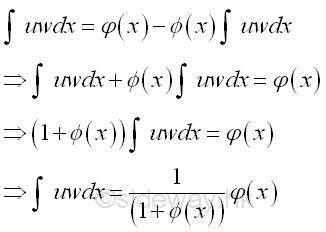6. If the integral is a power of sin or cos function in which the power is positive integer.

The techniques 4 and 5 of integration by parts can be applied.

1.  power of sin function in which the power is positive integer, imply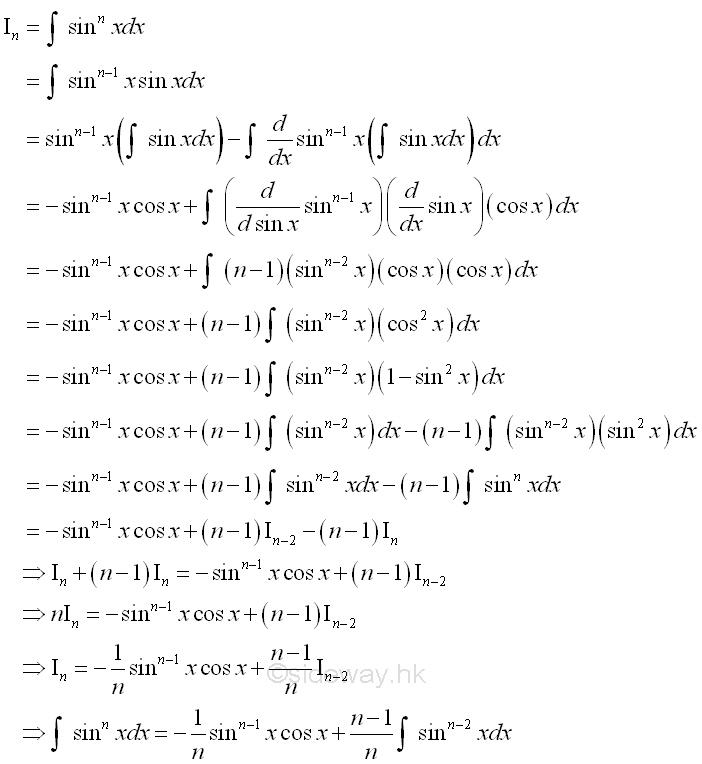2.  power of cos function in which the power is positive integer, imply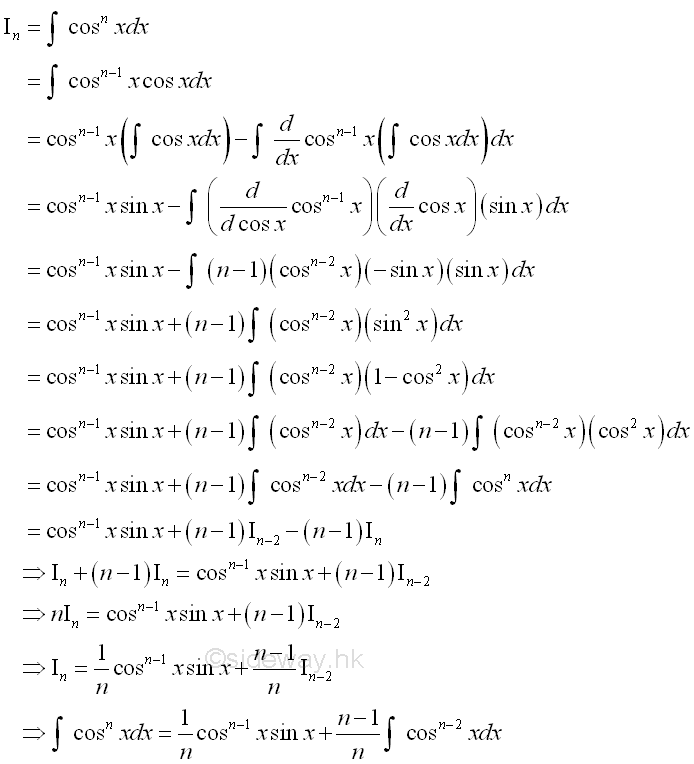ID: 111000034 Last Updated: 10/30/2011 Revision: 0 Ref:References

1. S. James, 1999, Calculus
2. B. Joseph, 1978, University Mathematics: A Textbook for Students of Science &amp; EngineeringHome 5

Management

HBR 3

Information

Recreation

Culture

Chinese 1097

English 339

Computer

Hardware 224

Software

Application 213

Latex 52

Manim 204

KB 1

Numeric 19

Programming

Web 289

Unicode 504

HTML 66

CSS 65

SVG 46

ASP.NET 270

OS 429

Python 72

Knowledge

Mathematics

Algebra 84

Geometry 33

Calculus 67

Engineering

Mechanical

Rigid Bodies

Statics 92

Dynamics 37

Control

Natural Sciences

Electric 27